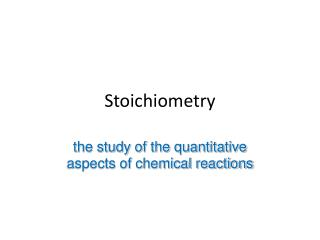# Stoichiometry - PowerPoint PPT PresentationDownload PresentationStoichiometry

StoichiometryDownload Presentation## Stoichiometry

- - - - - - - - - - - - - - - - - - - - - - - - - - - E N D - - - - - - - - - - - - - - - - - - - - - - - - - - -
##### Presentation Transcript

1. Stoichiometry the study of the quantitative aspects of chemical reactions

2. Calculate the percentage composition by mass of the following compounds Na2S (NH4)2S

3. The Mole • A counting unit • Similar to a dozen, except instead of 12, it’s 602 billion trillion 602,000,000,000,000,000,000,000 • 6.02 X 1023 (in scientific notation) • This number is named in honor of Amedeo Avogadro (1776 – 1856), who studied quantities of gases and discovered that no matter what the gas was, there were the same number of molecules present

4. Just How Big is a Mole? • Enough soft drink cans to cover the surface of the earth to a depth of over 200 miles. • If you had Avogadro's number of unpopped popcorn kernels, and spread them across the United States of America, the country would be covered in popcorn to a depth of over 9 miles. • If we were able to count atoms at the rate of 10 million per second, it would take about 2 billion years to count the atoms in one mole.

5. Everybody Has Avogadro’s Number!But Where Did it Come From? • It was NOT just picked! It was MEASURED. • One of the better methods of measuring this number was the Millikan Oil Drop Experiment • Since then we have found even better ways of measuring using x-ray technology

6. 1 dozen cookies = 12 cookies • 1 mole of cookies = 6.02 X 1023 cookies • 1 dozen cars = 12 cars • 1 mole of cars = 6.02 X 1023 cars • 1 dozen Al atoms = 12 Al atoms • 1 mole of Al atoms = 6.02 X 1023 atoms Note that the NUMBER is always the same, but the MASS is very different! Mole is abbreviated mol

7. A Mole of ParticlesContains 6.02 x 1023 particles = 6.02 x 1023 C atoms = 6.02 x 1023H2O molecules = 6.02 x 1023NaCl formula units 6.02 x 1023 Na+ ions and 6.02 x 1023Cl– ions 1 mole C 1 mole H2O 1 mole NaCl

8. Grams ↔ moles ↔ particles • How many moles of Na are in 42 g of Na?

9. What is the mass of 0.28 mol of iron?

10. How many moles are in 1.0 x 109 atoms?

11. How many atoms are in 7.2 mol of chlorine?

12. How many atoms are in 36 g of bromine?

13. What is the mass of 1.20 x 1025 atoms of sulfur?

14. What is the mass of 0.25 mol of Fe2O3?

15. How many molecules are there in 52 g of CO?

16. What is the mass of 3.01 x 1023 formula units of Fe2O3?

17. How many moles of O atoms do you have when you have 1.20 x 1025 N2O5 molecules?

18. How many H atoms are in 17.5 g (NH4)2C2O4?